## Sunday, July 22, 2007

### Irodov Problem 1.42

(a) From the basic problem definition,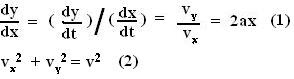Thus, we can calculate the velocity of the particle from (1) and (2) as,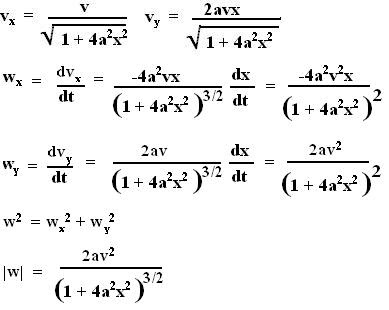we can now find |w| at x=0, as 2av2

The radius of curvature can be calculated mathematically from the equation of the curve or by using centripetal acceleration.

Using the formula for radius of curvature: We know that,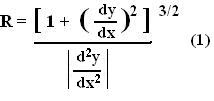see here for explanation.

Thus, for our case,The alternative method using centripetal acceleration is given below: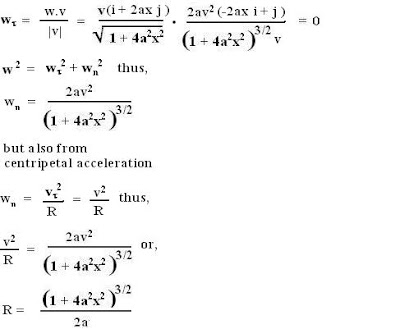Actually the fact that the magnitude of velocity is not changing, that implies that the tangential acceleration is 0, nevertheless we derived the same obvious result above.

(b) This part is the same as above only the curve is different. Differentiating both sides of the equation of the ellipse,Now as done before in part (a), from (2),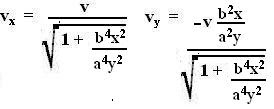Now following the same procedure as we did in part (a),At x=0, y=+ or - b. Substituting these values above we obtain,As in part (a) we can calculate the radius of curvature using both the methods. We further also know that that the tangential acceleration will be 0 since there is no tangential acceleration. The centripetal acceleration must thus be equal to the accelaration derived above. For this part I will only solve using the centripetal method since its simpler. Thus we have,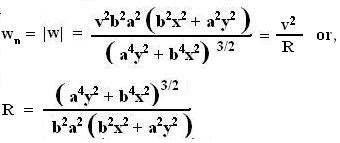Now at x=0, we can determine the radius of curvature as, a2/b.